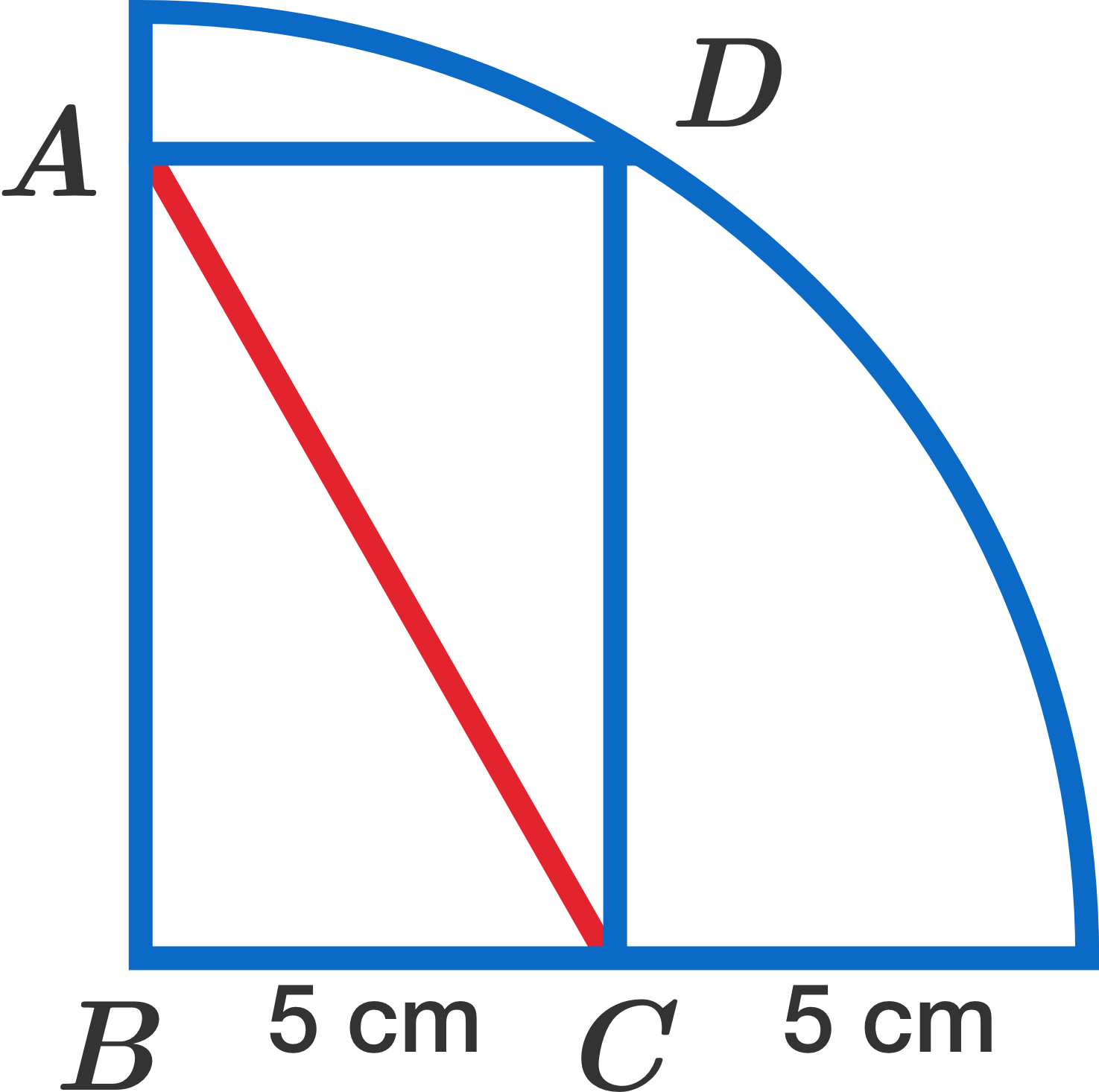# Arc And Lines

Geometry Level 1A rectangle $ABCD$ is inscribed in a quarter circle. If point $B$ is the center of the circle, find the length of $AC$ (in cm).

×